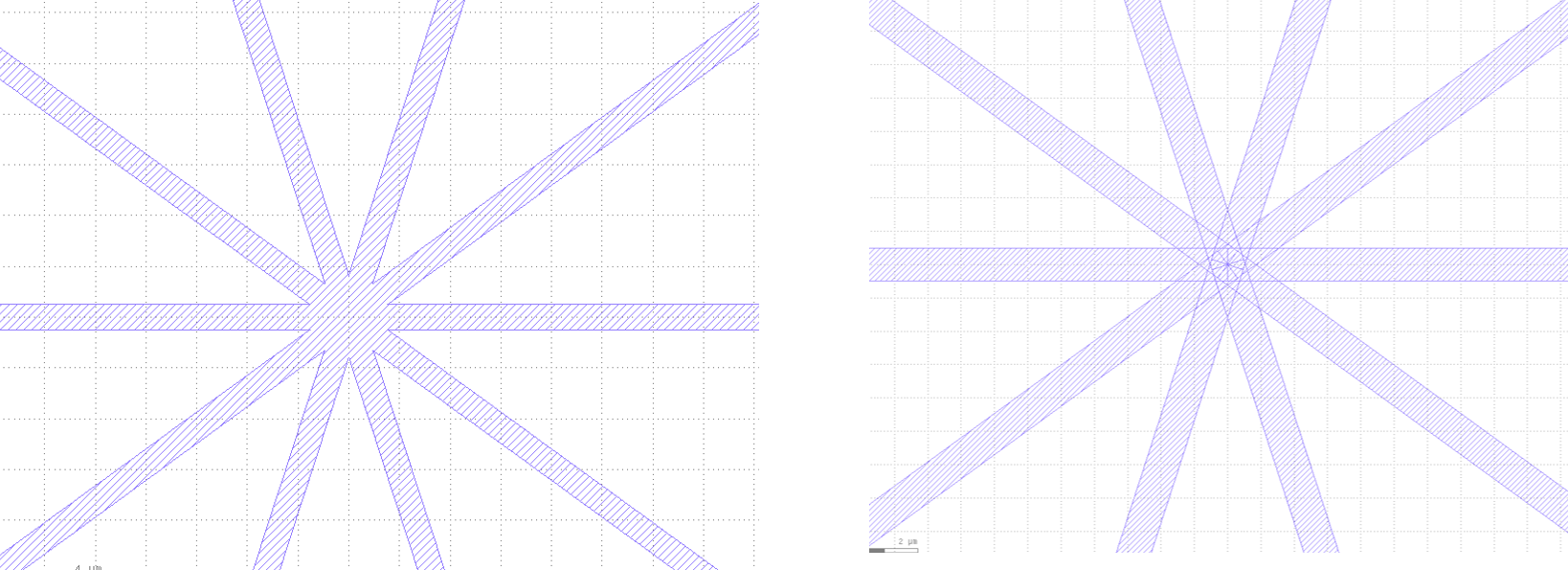Home Forums Nazca Questions and Answers Merge two structures

Viewing 6 posts - 1 through 6 (of 6 total)
• Author
Posts
• #6622

Dear Ronald and Nazca team,

I would like to merge two structures so that they don’t appear as two separate structures in the gds (for example the ones below):

``````nd.strt(length=20, width=1, layer=3).put()
nd.export_gds(filename='str_and_bend')``````

One can definitely do it in KLayout but it would be nice if there was a way to do it in Nazca itself, especially this would be a great help for dense structures.

Thanks,
Neetesh

#6628

Dear Neetesh,

Would putting the structures in a separate cell solve your problem? E.g.:

```import nazca as nd

with nd.Cell("strt_bend") as strt_bend:
nd.strt(length=20, width=1, layer=3).put()

for i in range(10):
strt_bend.put(0, 0, i * 36)

nd.export_gds()
```

Xaveer

#6629

Dear Xaveer,

This is a nice example. So I would like to do something like the left figure instead of the one on the right (which you get from your example above).
Best,
Neetesh#6632

Dear Neetesh,

The picture helps, thanks. This can be approached analogous to the thread remove holes.

Note that if you intend to draw just polygon shapes rather than circuit elements, it is better to use nd.Polygon() objects in Nazca and the nd.geometries module for geometry helper functions, instead of nd.strt() and nd.bend() structures. In the former case, one can manipulate polygons directly with the nd.pyclipper module. Also check out the merge_polygons function in the inverted MMI tutorial.

In the “bend and strt” case you first have to pry the polygons out of the bend or strt cells. Note also that bends and strt are circuit elements, which have a much larger overhead associated with them. This becomes more noticeable when you use (very) large numbers of them.

Ronald

#6645

In the case of merging polygons in a cell, the cell_iter() can help out, like described in the example below. The merge_cell_polygons function returns a new cell with the merged result, although the pyclipper may not handle all cases as desired for GDS.

``````
import nazca as nd
from collections import defaultdict

def merge_cell_polygons(cell):
"""Flatten a cell and merge polygons per layer.

Args:
cell (Cell): cell to flatten and merge polygon of.

Returns:
Cell: flattened cell with merged polygons per layer.
"""
layerpgons = defaultdict(list)
for P in nd.cell_iter(cell, flat=True):
if P.cell_start:
for pgon, xy, bbox in P.iters['polygon']:
layerpgons[pgon.layer].append(xy)
with nd.Cell(name=f"{cell.cell_name}_merged") as C:
for layer, pgons in layerpgons.items():
merged = nd.clipper.merge_polygons(pgons)
for pgon in merged:
nd.Polygon(points=pgon, layer=layer).put(0)
return C

with nd.Cell('test') as my_cell: